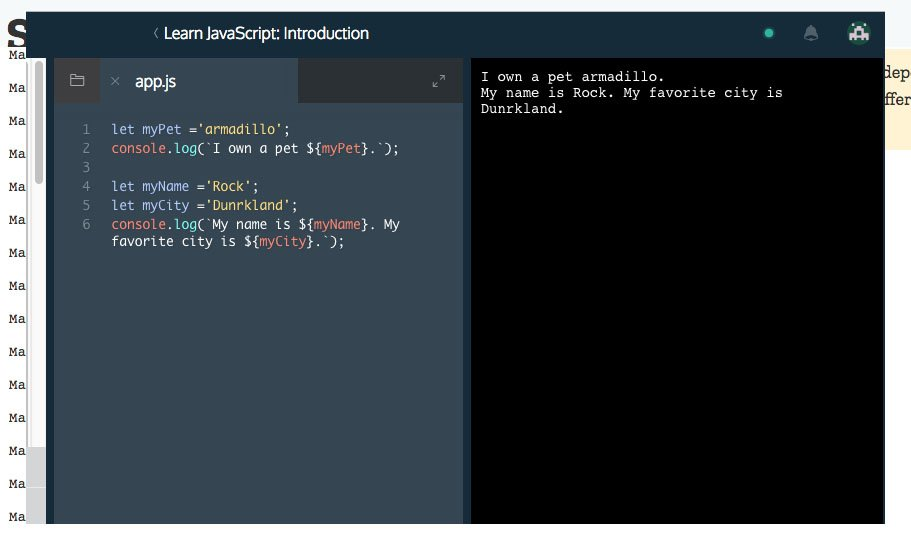# Learn JavaScript Journey Part 2

It is part two or day two on JavaScript learning. So far so good. Of course everything here is just and introduction.So in todays lesson we will continue learning JavaScript on Codeacademy.com. It will be part 2 of the introduction course.

### Variables

• Variables hold reusable data in a program.

Variables are used to write code that is easy to understand and repurpose. Variables allow us to assign data to a word and use the word to reference the data. If the data changes ( we can replace the variable’s value instead of re-writing the program.

```// This line of code sets the variable location to the string New York City
const location = 'New York City';

// This line of code sets the variable latitude to the number 40.7
let latitude = 40.7;

// This line of code sets the variable inNorthernHemisphere to true
let inNorthernHemisphere = true;

console.log(location);
console.log(latitude);
console.log(inNorthernHemisphere);```

Output:

```New York City
40.7
true
```

### Variable: const

• JavaScript will throw an error if you try to reassign `const` variables.

This how you declare a constant variable or a const:

1. `const`, short for constant, is a JavaScript keyword that creates a new variable with a value that cannot change.
2. Notice that the MyName word has no spaces, and we capitalised the `N`. Capitalising in this way is a standard convention in JavaScript called camelCasing, because the capital letters look like the humps on a camel’s back.
```const myName = 'Arya';
console.log(myName);
// Output: Arya```

As for this exercise these are an examples of const variables. In the last line we try to assign value to a constant variable named entree. As you can see below in the output you immediately get an error.

```const myName = 'Arya';
console.log(myName);

const myAge = 11;
console.log(myAge);

const price = 12;
console.log(entree, price);
entree = 'Tacos';```

Output:

```Arya
11
/home/ccuser/workspace/learn-javascript-variables-const/app.js:10
entree = 'Tacos';
^

TypeError: Assignment to constant variable.
at Object.<anonymous>```

### Variable: let

Let variables different from const can be reassigned.

• You can reassign variables that you create with the `let` keyword.

In the example above, the `let` keyword is used to create the `meal` variable with the string `'Enchiladas'` saved to it. On line three, the `meal` variable is changed to store the string `'Tacos'`.

```let meal = 'Enchiladas';
console.log(meal);
meal = 'Tacos';
console.log(meal);

let changeMe = true;
console.log(changeMe);
changeMe = false;
console.log(changeMe);
```

Output:

```Enchiladas
Tacos
true
false
```

### Undefined variables

• Unset variables store the primitive data type `undefined`.

If you do not set value to your variable. JavaScript creates space for this variable in memory and sets it to `undefined`Undefined is the fifth and final primitive data type. JavaScript assigns the undefined data type to variables that are not assigned a value. For example.

```let whatAmI;
console.log(whatAmI);
let notDefined;
console.log(notDefined);
let valueless;
console.log(valueless);```

Output:

```undefined
undefined
undefined```

### Mathematical Assignment Operators

• Mathematical assignment operators make it easy to calculate a new value and assign it to the same variable.

There are lots of different ways to write code. But it is always best to write it in most efficient way.

1. The operators (`+=``-=`,`/=` and `*=`) perform the mathematical operation of the first operator (`+``-`,`/` or `*`) using the number on the right, then assign the new value to the variable.
2. These operators are increment (`++`) and decrement (`--`) operators. These operators are responsible for increasing and decreasing a number variable by one, respectively.

As in the example below using mathematical operator += we can add value 1 to the variable without having to write the whole variable again.

```let molecule1 = 16;
let molecule2 = 16;

// Add and assign to molecule below
molecule1 += 1;
console.log('molecule1:',molecule1);
molecule2 = molecule2 + 1;
console.log('molecule:',molecule2);```

Output:

```molecule1: 17
molecule2: 17```

In this example. We will increase molecule value by 16. Will multiply particle by 6.02. And increase the value of assay by 1.

```let molecule = 16;
let particle = 18;
let assay = 3;
console.log(molecule,particle,assay)
console.log('After:')
// Add and assign to molecule below
molecule += 16;

// Multiply and assign to particle below
particle *= 6.02;

// Increment assay by 1
assay ++;
console.log(molecule,particle,assay)```

Output:

```16 18 3
After:
32 108.35999999999999 4```

### String Interpolation

• The `+` operator is used to interpolate (combine) multiple strings.

The JavaScript term for inserting the data saved to a variable into a string is string interpolation.

The `+` operator, known until now as the addition operator, is used to interpolate (insert) a string variable into a string, as follows:

```let myPet = 'armadillo';
console.log('I own a pet ' + myPet + '.')
let favoriteAnimal = 'dog';
console.log('My favourite animal ' + favoriteAnimal + '.')```

Output:

```I own a pet armadillo.
My favourite animal dog.```

## String Interpolation II

• In JavaScript ES6, backticks (`) and `\${}` are used to interpolate values into a string.

In the newest version of JavaScript (ES6) we can insert variables into strings with ease, by doing two things:

1. Instead of using quotes around the string, use backticks (this key is usually located on the top of your keyboard, left of the `1` key).
2. Wrap your variable with `\${myVariable}`, followed by a sentence. No `+`s necessary.

ES6 string interpolation is easier than the method you used last exercise. With ES6 interpolation we can insert variables directly into our text.

```let myPet ='armadillo';
console.log(`I own a pet \${myPet}.`);

let myName ='Rock';
let myCity ='Dunrkland';
console.log(`My name is\${myName}. My favorite city is \${myCity}`);```

Output:

```I own a pet armadillo.
My name isRock. My favorite city is Dunrkland```

This is the end of this part 2.

Go to first 1 part <<

Go to the third 3 part >>